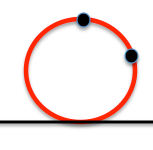# Metric geometry : Problema fundamental de tangencias : PPrClassically tangencies problems have been studied looking geometric constructions of each case study.

Concepts power of a point on a circle can address the problems with a unifying approach, so that any tangency or incidences statement generally be reduced to a more generic fundamental problem tangents denominate (PFT).

The PFT can be stated as the problem of determining a circle passing through two points and is tangent to a line or to another circle.

A higher level of abstraction would replace items passing through a condition of belonging to a beam, although this approach will leave it pending for later.

We will solve the first case study stating the problem as:

Determine the circles through the points A and B and the line tangent to r

## Analysis of the fundamental problem of tangents

In figure analysis shows that the circumference c center C can be one solution to the problem as it passes through points A and B and is tangent to the line r. In this figure ,in which the circumference represent solution we are looking for, we can determine properties that serve to derive a construction that allows us to determine it.

The line through the points A and B the short straight r at a point P. The potency of this point on the circumference is:

From the above expression we deduce that if we get the segment value PT (Power root) we get the point T tangent and the problem reduces to determining the circle through three points: A, B and T (its center will be at the intersection of two bisectors).

## Resolving the problem.

Determine the value of the power by one of the constructions used to solve proportional means:

As the power point P with respect to any circle through the points A and B is the same, We can use an auxiliary circle of any radius passing through these points, as shown in the center figure O1, located on the bisector of A and B.

The power value obtaining determine the tangent segment from P this auxiliary circle; for this, build a arc able 90 grados the segment PO1

The segment value tangency ( P-T1) take it on the line r to determine the point T of tangency with a simple twist of center P.

## Solution Number

Depending on the direction in which we take the segment PT get one or other of the two possible solutions.

Metric geometry